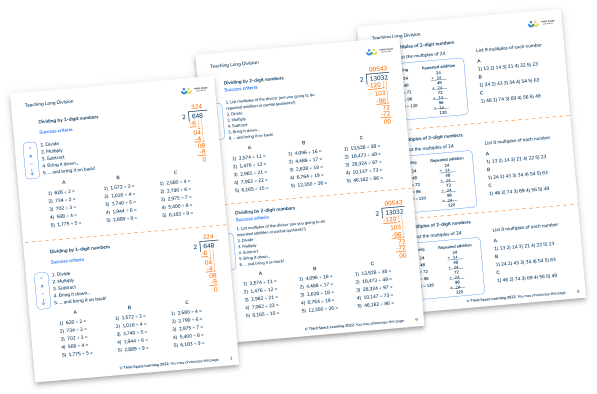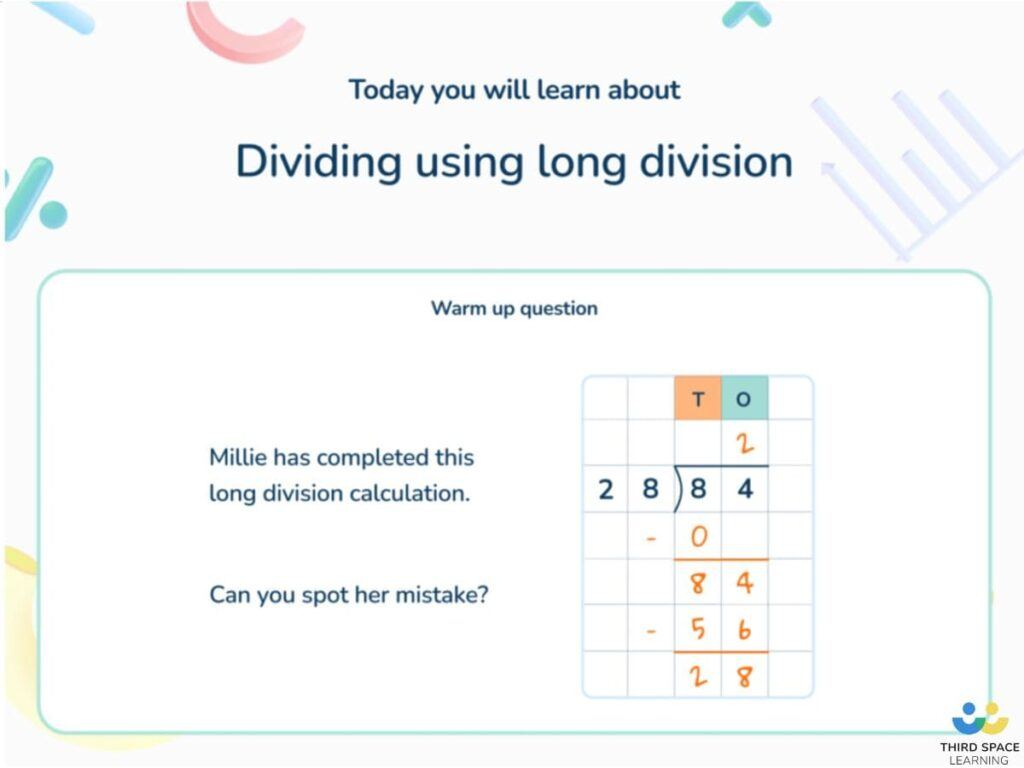Math teaching support you can trustone-on-one tutoring sessionsschools supported

[FREE] Thanksgiving Math Activities

10 fun Thanksgiving math activities for all your elementary students. Includes printable resources!

# Division Questions That Will Test Your Elementary School Students

Division questions will appear throughout elementary school as division is one of the four mathematical operations- addition, subtraction, multiplication and division. Children will encounter all four operations from as early as third grade. However, concepts of division such as sharing are exposed to children as early as kindergarten.

From experience, children seem to find division the trickiest to grasp. Here are some questions to use for division practice with children, from basic division to tricky word problems to challenge your student’s division skills, plus answer keys and expert guidance on how to solve them.

### What are division questions?

Division questions can be standard arithmetic (e.g. 25 ÷ 5 or 3,426 ÷ 2) or can be presented as division word problems which present a math problem in a ‘real-life’ context.

In this case of division, this will involve sharing or equal grouping and may require children to interpret the remainders appropriately, either by rounding up or down.

### How to solve division questions

In a division problem:

• The amount being divided is called the dividend
• Whatever the dividend is being divided by is called the divisor;
• The answer is called the quotient.

Division questions may be solvable mentally, perhaps by recalling multiplication facts or place value knowledge to divide into equal groups. For example:

a) Mrs. Patel has 16 candies to share between 4 children. How many should each child get?

16 candies ÷ 4 = 4 candies each. Children should be able to use their multiplication and division fact families to solve this.

b) A $283 bill at a restaurant has to be split between 10 people. How much should each person pay?$283 ÷ 10 = 28.30 each. Children should be able to use their place value knowledge for this. Other division questions may require the use of a formal written method, such as the partial product method. c) A length of ribbon measures 28.8cm. It is cut into 6 equal pieces. How long is each piece? 28.8cm ÷ 6 = 4.8cmTeaching Long Division Worksheets Division can be tough, but it doesn't have to be! Download this free worksheet to help your students better understand long division specifically.Download Free Now! ### Division questions elementary Division questions will appear throughout elementary school. However, as the formal method of division (commonly known as the partial product method) isn’t introduced until upper elementary school, the division questions students will encounter will reflect this. In 3rd grade, students will fluently divide within 100 relating division to their multiplication facts. By 4th grade, students will divide up to four-digit whole number dividends by one-digit divisors using strategies based on place value, such as partial quotients, area models, and arrays. Students in 4th grade should also work with division of whole numbers involving remainders. By 5th grade, students will divide up to four-digit whole number dividends by two-digit divisors using strategies based on place value. Students at this level will also begin to divide decimals to the hundredths place with up to four-digit dividends and two-digit divisors using similar strategies. The standard algorithm for division (also known as long division) is introduced at different times based on what standards your state follows. Common Core states that students should fluently divide multi-digit numbers using the standard algorithm in 6th grade. TEKS states that students start to use the standard algorithm in 4th grade. See below the table, which has been adapted from the Common Core and aligns with TEKS as well, that details the development of skills in division throughout elementary school. If you’re looking for more information on how to teach division or how to fill student’s division knowledge gaps using interventions, read our articles written by math specialists.Example of a Third Space Learning tuition slide on the partial quotient method ### Division questions for 3rd grade 1. 75 children take part in a game. There are 5 children on each team. How many teams are there altogether? Answer: 45 children ÷ 5 = 9 teams 2. David wants to decorate cakes. Each cake will have 3 cherries. David has 18 cherries. How many cakes can he decorate? Answer: 18 cherries ÷ 3 = 6 cakes 3. John needs 48 balloons. The store sells balloons in packs of 6. How many packs does he need to buy? Answer: 48 balloons ÷ 6 = 8 packs ### Division questions for 4th grade 1. Riley works at an apple orchard and is putting 496 apples into bags. Each bag holds 4 apples. How many bags of apples can she make? Answer: 496 apples ÷ 4 = 124 bags of apples 2. Bryan made4,932 selling albums at a concert. Each album was sold for $9. How many albums did he sell? Answer:$4,932 ÷ 9 = 548 albums

3. Sam wants to walk a long distance, for charity, over 6 weekends. The total distance Sam wants to walk is 293km. Approximately how far should he walk each weekend?

Answer: 293km ÷ 6 = 48r5 km. We can round this up to 49km – if he walks 49km each weekend he will definitely meet his target!

### Division questions for 5th grade

1. I have 1 ½  liters of juice. I need to share it all equally between 6 glasses. How many milliliters of juice should I pour into each glass?

Answer: 1 ½ liters = 1,500ml. 1,500ml ÷ 6 = 250ml of juice in each glass

2. A school fair raises $5,168. The school keeps ¼ of the money for new playground equipment. It gives the rest to charity. How much money does it give to charity? Answer:$5,168 ÷ 4 = $1,292 kept by the school. They give the rest to charity, which is$5,168 – $1,292 =$3,876 to charity

3. A charity organization raised $4,743 at their monthly fundraiser. They share this equally amongst their 12 branches. How much does each branch get? Answer:$4743 ÷ 12 = $395.2 ### Division Questions for 6th grade 1. 8827 ÷ 97 Answer: 91. The multiples here could be listed more quickly (than by using repeated addition or partitioning) by adding 100 and subtracting 3 each time. 2. 3 pineapples cost the same as 2 mangoes. One mango costs$1.35. How much does one pineapple cost?

Answer: 2 mangoes = $1.35 x 2 =$2.70 (the same as 3 pineapples). $2.70 ÷ 3 = 90¢, which is the cost of 1 pineapple. 3. Amina posts three large letters. The postage costs the same for each letter. She pays with a$20 note. Her change is $14.96. What is the cost of posting one letter? Answer:$20 – $14.96 =$5.04 (the cost of three letters). $5.04 ÷ 3 =$1.68, which is the cost of one letter.

### Simple division questions

1. 8 meters of rope is cut into 4 equal lengths to make jump ropes. How long is each jump rope?

Answer: 8m ÷ 4 = 2m

2. Circle two numbers which divide by 5 with no remainder: 9, 50, 12, 35, 31, 57

Answer: 50 and 35 – both are multiples of 5 as they end in either a 0 or 5

3. 28 children go swimming in groups of 4. How many groups will there be?

Answer: 28 children ÷ 4 = 7 groups

### Difficult division questions

1. 999 will divide exactly by 37. There is no remainder.

a) Write down the remainder when 1000 is divided by 37.

b) Write down the remainder when 998 is divided by 37.

c) Write down a multiple of 37 that is greater than 1,000.

a) As 1,000 is only 1 more than 999, the remainder will be 1.

b) As 998 is only 1 less than 999, the remainder will be 1 less than the divisor (37), so the remainder is 36.

c) As 999 is a multiple of 37, if we add 37 we will get another multiple of 37. We can work out that 999 + 37 = 1,036, which is a multiple of 37 greater than 1,000

2. Write the missing number: 12.5 ÷ ___ = 7.5 ÷ 1.5

Answer: 7.5 ÷ 1.5 = 5 – we can figure this out by counting in multiples of 1.5 until we reach 7.5 (5 of 1.5 is 7.5). We could also make each number 10 times bigger to make it ‘easier’ to solve as the answer will be the same: 75 ÷ 15 = 5.

We are now left with 12.5 ÷ ___ = 5. When the divisor is missing, we can divide the dividend by the quotient instead to reach the answer. 12.5 ÷ 5 = 2.5, so the missing number is 2.5.

3. A bus company has 62 buses. On average, each bus travels 19 miles on a gallon of fuel and goes 284 miles each day. The bus company says it needs about 900 gallons of fuel every day. Make an estimate to show whether what the company says is reasonable.

Answer: To make an estimate, we could round 19 miles to 20 and 284 miles to 280. 280 miles per day ÷ 20 miles per gallon = 14 gallons per bus per day.

For approximately 60 buses (we’ve rounded 62 to 60), this would be 14 x 60, which would be 840 gallons. This is close to 900 gallons so the company is fairly accurate.

Looking for more resources to support your teaching of division? See our blog on printable division worksheets.

Do you have students who need extra support in math?
Give your students more opportunities to consolidate learning and practice skills through personalized math tutoring with their own dedicated online math tutor.

Each student receives differentiated instruction designed to close their individual learning gaps, and scaffolded learning ensures every student learns at the right pace. Lessons are aligned with your state’s standards and assessments, plus you’ll receive regular reports every step of the way.

Personalized one-on-one math tutoring programs are available for:

The content in this article was originally written by primary school teacher Sophie Bartlett and has since been revised and adapted for US schools by elementary math teacher Jaclyn Wassell.##### Sophie Bartlett
Year 6 Teacher
Sophie teaches mixed age classes at a small school in central England. She is a self confessed grammar pedant and number nerd so we've welcomed her as a regular author and editor for Third Space Learning.
x#### Teaching Long Division Worksheets